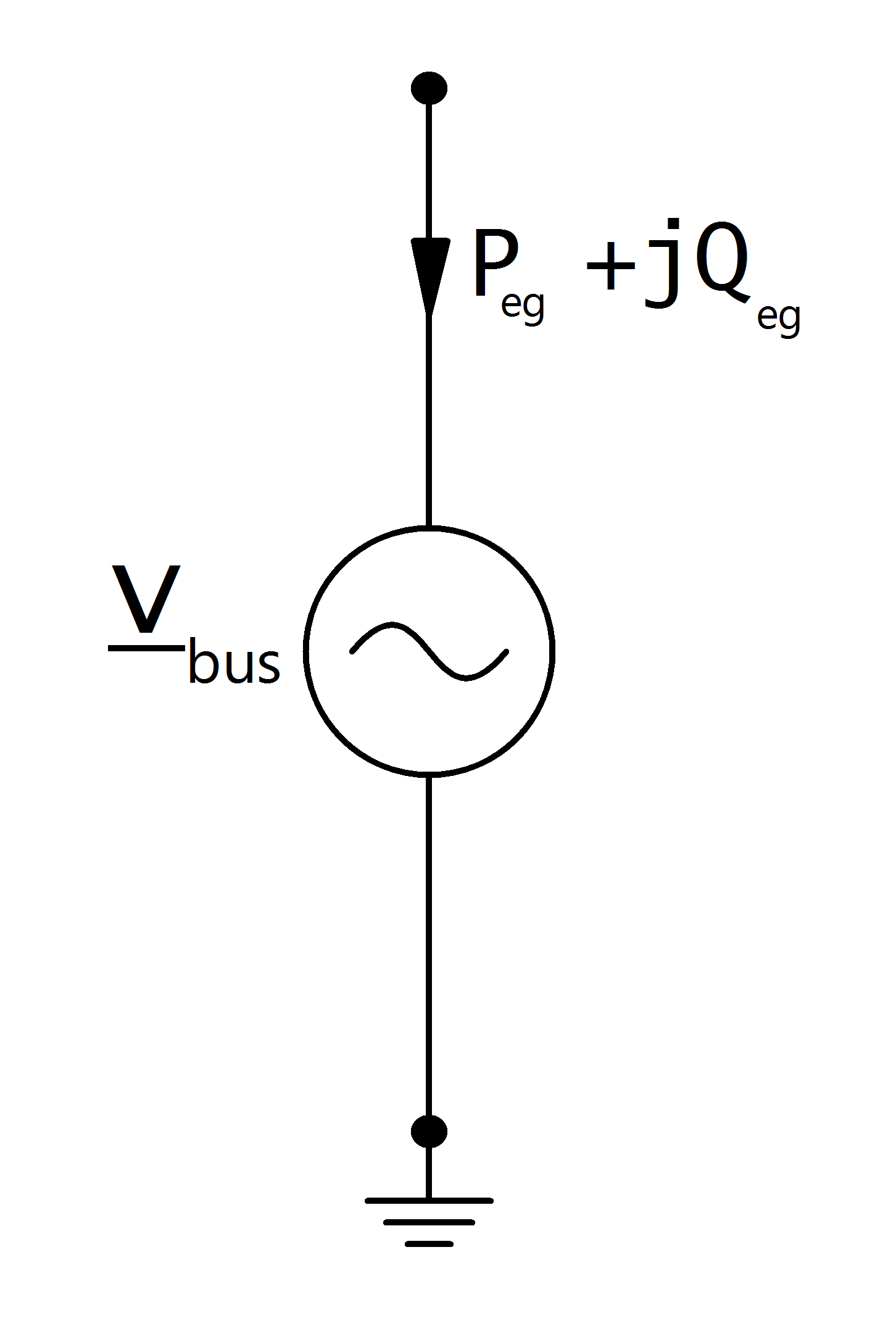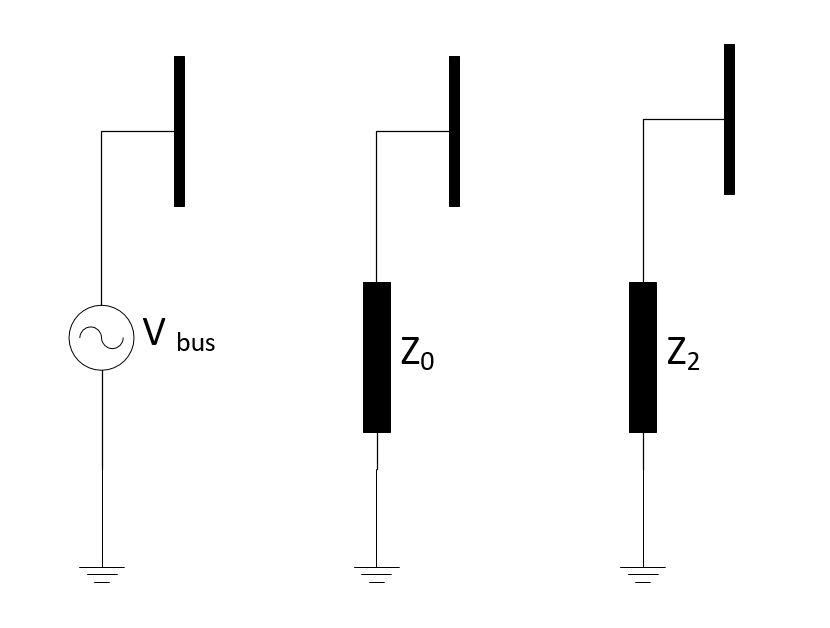# External Grid

Note

Power values of external grids are given in the generator system, therefore p_mw is negative if the external grid is absorbing power and positive if it is supplying/generating power.

## Create Function

pandapower.create.create_ext_grid(net, bus, vm_pu=1.0, va_degree=0.0, name=None, in_service=True, s_sc_max_mva=nan, s_sc_min_mva=nan, rx_max=nan, rx_min=nan, max_p_mw=nan, min_p_mw=nan, max_q_mvar=nan, min_q_mvar=nan, index=None, r0x0_max=nan, x0x_max=nan, controllable=nan, slack_weight=1.0, **kwargs)

Creates an external grid connection.

External grids represent the higher level power grid connection and are modelled as the slack bus in the power flow calculation.

INPUT:

net - pandapower network

bus (int) - bus where the slack is connected

OPTIONAL:

vm_pu (float, default 1.0) - voltage at the slack node in per unit

va_degree (float, default 0.) - voltage angle at the slack node in degrees*

name (string, default None) - name of of the external grid

in_service (boolean) - True for in_service or False for out of service

s_sc_max_mva (float, NaN) - maximal short circuit apparent power to calculate internal impedance of ext_grid for short circuit calculations

s_sc_min_mva (float, NaN) - minimal short circuit apparent power to calculate internal impedance of ext_grid for short circuit calculations

rx_max (float, NaN) - maximal R/X-ratio to calculate internal impedance of ext_grid for short circuit calculations

rx_min (float, NaN) - minimal R/X-ratio to calculate internal impedance of ext_grid for short circuit calculations

max_p_mw (float, NaN) - Maximum active power injection. Only respected for OPF

min_p_mw (float, NaN) - Minimum active power injection. Only respected for OPF

max_q_mvar (float, NaN) - Maximum reactive power injection. Only respected for OPF

min_q_mvar (float, NaN) - Minimum reactive power injection. Only respected for OPF

r0x0_max (float, NaN) - maximal R/X-ratio to calculate Zero sequence internal impedance of ext_grid

x0x_max (float, NaN) - maximal X0/X-ratio to calculate Zero sequence internal impedance of ext_grid

slack_weight (float, default 1.0) - Contribution factor for distributed slack power flow calculation (active power balancing)

** only considered in loadflow if calculate_voltage_angles = True

controllable (bool, NaN) - True: p_mw, q_mvar and vm_pu limits are enforced for the ext_grid in OPF. The voltage limits set in the ext_grid bus are enforced.

False: p_mw and vm_pu setpoints are enforced and limits are ignored. The vm_pu setpoint is enforced and limits of the bus table are ignored. defaults to False if “controllable” column exists in DataFrame

EXAMPLE:

create_ext_grid(net, 1, voltage = 1.03)

create_ext_grid(net, 1, voltage=1.03, s_sc_max_mva=1000, rx_max=0.1, r0x0_max=0.1, x0x_max=1.0)

## Input Parameters

net.ext_grid

 Parameter Datatype Value Range Explanation name string name of the external grid bus* integer index of connected bus vm_pu* float $$>$$ 0 voltage set point [p.u] va_degree* float angle set point [degree] max_p_mw** float Maximum active power min_p_mw** float Minimum active power max_q_mvar** float Maximum reactive power min_q_mvar** float Minimum reactive power s_sc_max_mva*** float $$>$$ 0 maximum short circuit power provision [MVA] s_sc_min_mva*** float $$>$$ 0 minimum short circuit power provision [MVA] rx_max*** float 0…1 maxium R/X ratio of short-circuit impedance rx_min*** float 0…1 minimum R/X ratio of short-circuit impedance r0x0_max**** float 0…1 maximal R/X-ratio to calculate Zero sequence internal impedance of ext_grid x0x_max**** float 0…1 maximal X0/X-ratio to calculate Zero sequence internal impedance of ext_grid in_service* boolean True / False specifies if the external grid is in service.

*necessary for executing a power flow calculation
**optimal power flow parameter
***short-circuit calculation parameter
****Single phase short circuit/Three Phase load flow calculation parameters

## Electric Model

** Balanced Load Flow** The external grid is modelled as a voltage source in the power flow calculation, which means the node the grid is connected to is treated as a slack node:with:

\begin{align*} \underline{v}_{bus} &= vm\_pu \cdot e^{j \cdot \theta} \\ \theta &= shift\_degree \cdot \frac{\pi}{180} \end{align*}

** Unbalanced Load Flow / Single phase short ciruit **

The external grid is modelled as a voltage source for positive sequence model, which means the node the grid is connected to is treated as a slack node. For zero sequence and negative sequence external grid impedance is calculated:\begin{align*} \underline{v}_{1} &= vm\_pu \cdot e^{j \cdot \theta} \\ \theta &= shift\_degree \cdot \frac{\pi}{180} \end{align*} \begin{align*} \underline{Z}_{0} &= c \cdot \frac{(vm \cdot e^{j \cdot \theta})^2}{S_{sc_mva}} \\ \theta &= shift\_degree \cdot \frac{\pi}{180} \end{align*} \begin{align*} \underline{v}_{bus} &= vm\_pu \cdot e^{j \cdot \theta} \\ \theta &= shift\_degree \cdot \frac{\pi}{180} \end{align*}

## Result Parameters

net.res_ext_grid

 Parameter Datatype Explanation p_mw float active power supply at the external grid [MW] q_mvar float reactive power supply at the external grid [MVar]

Active and reactive power feed-in / consumption at the slack node is a result of the power flow:

\begin{align*} p\_mw &= P_{eg} \\ q\_mvar &= Q_{eg} \end{align*}

net.res_ext_grid_3ph

 Parameter Datatype Explanation p_a_mw float active power supply at the external grid : Phase A [MW] q_a_mvar float reactive power supply at the external grid : Phase A [MVar] p_b_mw float active power supply at the external grid : Phase B [MW] q_b_mvar float reactive power supply at the external grid : Phase B [MVar] p_c_mw float active power supply at the external grid : Phase C [MW] q_c_mvar float reactive power supply at the external grid : Phase C [MVar]

Active and reactive power feed-in / consumption at the slack node is a result of the power flow:

\begin{align*} p\_mw_{phase} &= P_{eg_{phase}} \\ q\_mvar_{phase} &= Q_{eg_{phase}} \end{align*}